## Anatomy of a GreEd Rule

 GreEd Introduction Anatomy of a GreEd Rule (this page) GreEd Tool Current Status Future Plans

GreEd treats a rule by breaking it down into its smallest possible parts. To understand how GreEd does this, let us take a look at a this simple rule: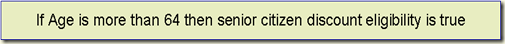The if and  then are common to all rules and therefore can be ignored in trying to identify elements that will need to be stored for each rule.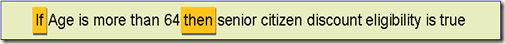This leaves us with the following: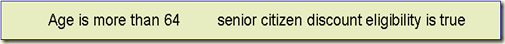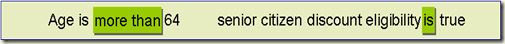These are nothing but two expressions, which can also be written as: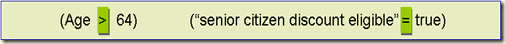In the above version “more than” is an operator represented as > and so “is” is represented by “=”

Now, can we further break down the expressions also into their constituent components?

We can, as you can see.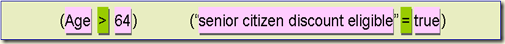We can parse out the things that the operators operate upon, also known as, operands. You will notice that for each expression, there is just one operator. This is true, not just for simple expressions but also complex ones. The firs expression is also called the Left Hand Side or LHS of a rule and the part of the rule on the right, is called, you guessed it, Right Hand Side or RHS. Note that the second expression is not an really an expression, but an assertion, meaning that if the condition on the left hand side is true, the one on the right side has to be true.

In effect, the operator is like a branch to which the operands are attached.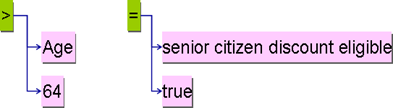So these are the elements we need to store for each expression in a rule.

Indeed, LISP, a language very popular amongst programming language enthusiasts and AI researchers also maintains a similar tree like structure.

In some cases, the order of the operands is important, e.g., in the case of “>” operator, the Age variable precedes the value ’64’. Therefore for such operators, the order of the operands needs to be saved too.

What about rules with slightly more complex expressions for their conditions. Let us add one more variable as part of the conditions for the rule: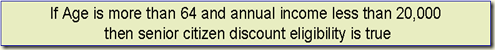Using the same understanding, this modified version of rule would be parsed as follows: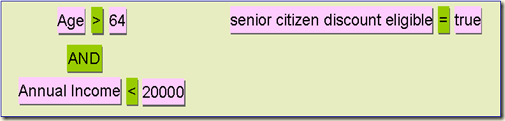The same rule represented as tree would look like this: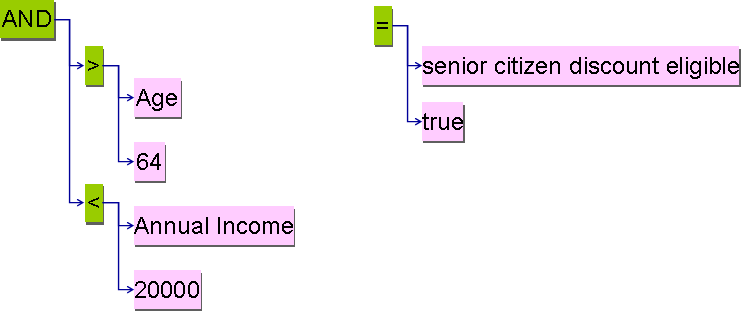Note that the left hand side still has only one expression;  just that now it is composed of two other expressions, one for age and another for annual income. Indeed, each of those sub-expressions can have other sub-expressions, allowing creation of expressions of tremendous complexity.

So, this is how rules are represented in GreEd, in a tree like structure, with each atomic part discretely savable.

## Advantages of GreEd’s Rule Structure

There are several advantages to having such a parsable structure:

1. Allow treating parts of rules as modular components, so that:
• they may be dragged and dropped
• new ones may be created
• existing ones could be reused in different rules
2. Ability to represent  rules in (or translate them into) most of the rule languages, since rules in all languages essentially have the same rule elements.
3. Since the terminal operands too are discrete elements they can be standard data elements which are accessed from a common data element repository based on a standard (ISO/IEC 11179). This gives the advantage of achieving interoperability with any other system that uses the same set of data elements annotated with concepts from ontologies/vocabularies. Not just syntactic but also semantic interoperability!